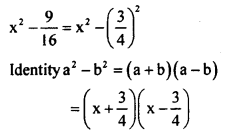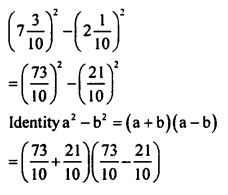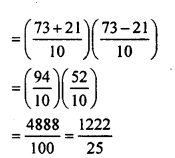# KSEEB Solutions for Class 8 Maths Chapter 4 Factorisation Ex 4.1

Students can Download Maths Chapter 4 Factorisation Ex 4.1 Questions and Answers, Notes Pdf, KSEEB Solutions for Class 8 Maths helps you to revise the complete Karnataka State Board Syllabus and score more marks in your examinations.

## Karnataka Board Class 8 Maths Chapter 4 Factorisation Ex 4.1

1. Resolve into factors.

Question i.
x² + xy
x² + xy = x(x + y)

Question ii.
3x² – 6x
3x² – 6x = 3x (x – 2)

Question iii.
(1.6)a² – (0.8)a
(1.6)² – (0.8)a
= (0.8 x 2a²) – (0.8)a
= 0. 8a(2a- 1)

Question iv.
5 – 10m – 20n
5 – 10m -20n = 5(1 – 2m – 4n)

2. Froctorise:

Question i.
a² + ax + ab + bx
a² + ax + ab + bx a(a + x) + b(a + x)
(a + x)(a + b)

Question ii.
3ac + 7bc – 3ad – 7bd
3ac + 7bc – 3ad – 7bd
c(3a + 7b – d(3a + 7b) (3a + 7b) (c-d)

Question iii.
3xy – 6zy – 3xt + 6zt
3y (x – 2z) – 3t(x – 2z)
(x – 2z) (3y – 3t)

Question iv.
y3 + 3y² + 2y – 6 – xy + 3x
y² (y – 3) + 2(y – 3) – x(y – 3)
(y- 3) (y² + 2 – x)

3. Factorise:

Question i.
4a² – 25
4a² – 25
= (2a)² – 52
[a² – b²=(a+b)(a-b)]
= (2a + 5)(2a – 5)

Question ii.
$$x^{2}-\frac{9}{16}$$
Ans.Question iii.
x4 – y4
x4 – y4
= (x²)² – (y²)²
Identity a² – b² = (a + b)(a – b)
= (x² + y²)(x² – y²)
= (x²+ y²)(x + y)(x – y)

Question iv.
$$\left(7 \frac{3}{10}\right)^{2}-\left(2 \frac{1}{10}\right)^{2}$$
Ans.Question v.
(0.7)² – (0.3)²
(0.7)² – (0.3)²
Identity a² – b² = (a + b)(a – b)
= (0.7 + 0.3)(0.7 – 0.3)
= (1.0)(0.4)
= 0.4

Question vi.
(5a-2b)²-(2a-b)²